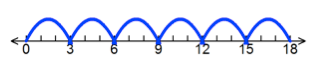Area Model

My Definition

Key Characteristics

The following are true of an area model:

• An area model is a rectangular diagram.

• An area model is a continuous model. Adjacent regions share a side.

• An area model is a visual representation of the multiplication and division of whole numbers, real numbers, and polynomials.

• An area model can be used as a pictorial model of the distributive property.

Example

Use the link below to further study examples of an area model.

Area Model Activity

Video Transcript (PDF)

Non-exampleRepeated addition on a number line to represent 6 x 3 is not an area model.

TEKS: 3(3)(G), 3(4)(E), 3(4)(K), 4(4)(C), 4(4)(E), 5(3)(D), 5(3)(F), 5(3)(I), 5(3)(J)